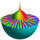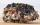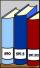# Roman numerals +

Add up the number written in Roman numerals. Write the results as a decimal number.

MMCCCLXXXIX + MCDXXXIX =  3828
MCMXCV + MCCLXXXVIII =  3283
CMXXVI + MMDCXCIV =  3620
MDXVII + MDIII =  3020
CCXLIX + MMCCL =  2499
CCII + MMCCCLXXII =  2574
DCCXCVIII + MMCXLI =  2939
CDXX + MMCCLXIX =  2689
CMLXVII + MDCCCLXI =  2828
MDXLIII + MMCDLIV =  3997

### Step-by-step explanation:

MMCCCLXXXIX + MCDXXXIX = 2389 + 1439 = 3828
MCMXCV + MCCLXXXVIII = 1995 + 1288 = 3283
CMXXVI + MMDCXCIV = 926 + 2694 = 3620
MDXVII + MDIII = 1517 + 1503 = 3020
CCXLIX + MMCCL = 249 + 2250 = 2499
CCII + MMCCCLXXII = 202 + 2372 = 2574
DCCXCVIII + MMCXLI = 798 + 2141 = 2939
CDXX + MMCCLXIX = 420 + 2269 = 2689
CMLXVII + MDCCCLXI = 967 + 1861 = 2828
MDXLIII + MMCDLIV = 1543 + 2454 = 3997Did you find an error or inaccuracy? Feel free to write us. Thank you!## Related math problems and questions:

• Roman numerals 2+Add up the number written in Roman numerals. Write the results as roman numbers.
• Roman numeralsWrite numbers written in Roman numerals as decimal.
• Roman numerals 2-Subtract up the number written in Roman numerals. Write the results as Roman numbers.Added together and write as decimal number: LXVII + MLXIV
• David numberJana and David train the addition of the decimal numbers so that each of them will write a single number and these two numbers then add up. The last example was 11.11. David's number had the same number of digits before the decimal point, the Jane's numbeAdd and write the result again as hours, minutes, seconds: 2hodiny45min15s + 1h20m50s =Add two mixed fractions: 2 4/6 + 1 3/6
• DozenWhat is the product of 26 and 5? Write the answer in an Arabic numeral. Add up the digits. How many of this is in a dozen? Divide #114 by this
• Written numberPlace+values x ten thousands =30 thousands
• Mistake in expressionWhile attempting to multiply the expression (2 - 5i)(5 + 2i) a student made a mistake. (2 - 5i)(5 + 2i) = 10 + 4i - 25i - 10i2 = 10 + 4(-1) - 25(-1) - 10(1) = 10 - 4 + 25 - 10 = 21 Complete the explanation and correct the error. Hint: The student inc
• Dividing by five and tenNumber 5040 divide by the number 5 and by number 10: a = 5040: 5 b = 5040: 10Add marks (+, -, *, /, brackets) to fullfill equations 1 3 6 5 = 10 This is for the 4th grade of the primary school - with no negative numbers yetOn the 4-th stop take on 56 and take off 38 passengers. How many were added (write as positive number) or shrunk (write as negative number) the count of passengers?April has 30 days. Roman saves his money in the box for the whole month. Every third day you saves 10 cents. How many cents did Roman saved during the whole month when start saving on April 1?Calculate: 2 . 1 + 0 . 10 + 7 . 10000 + 4 . 1000 + 6 . 100 + 0 . 100000 =Write decimal number 8.638333333 as a fraction A/B in the basic form. Given decimal has infinite repeating figures.Nicol mistake when calculate in school. Instead of add number 20 subtract it. What is the difference between the result and the right result?